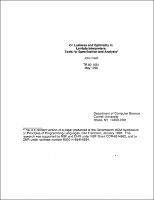## On Laziness and Optimality in Lambda Interpreters: Tools for Specification and Analysis##### Abstract
In this paper, we introduce a new formal system, $\Lambda CCL$, based on Curien's Categorical Combinators [Cur86a]. We show that $\Lambda CCL$ has properties that make it especially suitable for analysis and implementation of a wide range of $\lambda$-reduction schemes using shared environments, closures, or $\lambda$-terms. In particular, the term structure of $\Lamda CCL$ is very closely related to the structure of existing abstract machines for $\lambda$-reduction. $\Lambda CCL$ is powerful enough to mimic arbitrary (strong) reduction in the $\lambda$-calculus, yet in contrast to the systems in [Cur86a] it is also confluent (on ground terms).As an example of the practical utility of this formalism, we use it to specify a simple lazy interpreter for the $\lambda$-calculus, whose correctness follows trivially from the properties of $\LambdaCCL$. We then describe a labeled variant of $\Lambda CCL, \Lambda CCL^{L}$, which can be used as a tool to determine the degree of "laziness" possessed by various $\lambda$-reduction schemes. In particular, $\Lambda CCL^{L}$ is applied to the problem of optimal reduction in the $\lambda$-calculus. A reduction scheme for the $\lambda$-calculus is optimal if the number of redex contractions that must be performed in the course of reducing any $\lambda$-term to a normal form (if one exists) is guaranteed to be minimal. Results of Levy [Lev78, Lev80] showed that for a natural class of reduction strategies allowing shared redexes, optimal reductions were, at least in principle, possible. He conjectured that an optimal reduction strategy might be realized in practice using shared closures and environments as well as shared $\lambda$-terms. However, using $\Lambda CCL^{L}$, we show that the sharing allowed by environments and closures in $\Lambda CCL$ as implemented using standard term graph-rewriting techniques [BvEG$^{+}$87] is insufficient to implement optimal reduction.
1990-02
##### Publisher
Cornell University
##### Keywords
computer science; technical report
##### Previously Published As
http://techreports.library.cornell.edu:8081/Dienst/UI/1.0/Display/cul.cs/TR90-1091
technical report